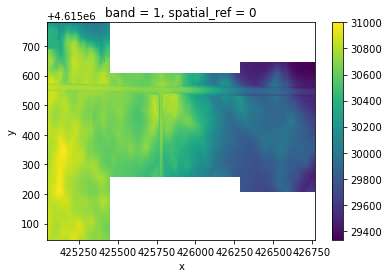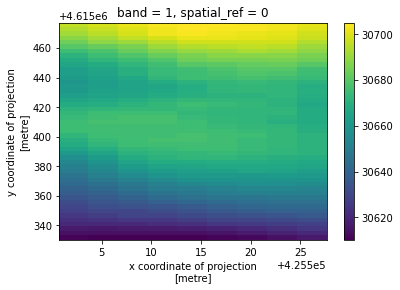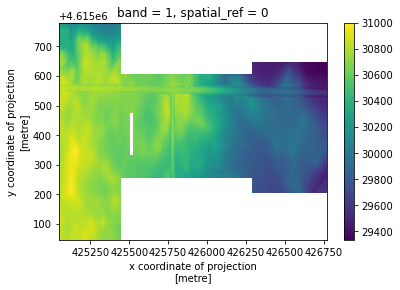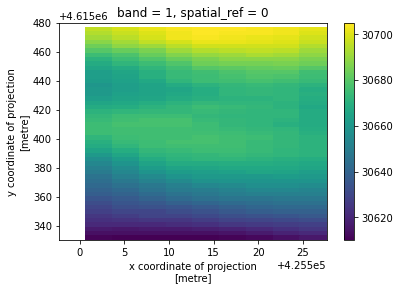# Example - Clip

API Reference for rio.clip:

:

import rioxarray

%matplotlib inline


See docs for rioxarray.open_rasterio

Notes:

• masked=True will convert from integer to float64 and fill with NaN. If this behavior is not desired, you can skip this.

:

xds = rioxarray.open_rasterio(
"../../test/test_data/compare/small_dem_3m_merged.tif",
)

:

xds.plot()

:

<matplotlib.collections.QuadMesh at 0x7fc957fb1ac0>## Clip using a geometry

By default, it assumes that the CRS of the geometry is the same as the CRS of the dataset. If it is different, make sure to pass in the CRS of the geometry.

:

geometries = [
{
'type': 'Polygon',
'coordinates': [[
[425499.18381405267, 4615331.540546387],
[425499.18381405267, 4615478.540546387],
[425526.18381405267, 4615478.540546387],
[425526.18381405267, 4615331.540546387],
[425499.18381405267, 4615331.540546387]
]]
}
]
clipped = xds.rio.clip(geometries)

:

clipped.plot()

:

<matplotlib.collections.QuadMesh at 0x7fc957829d90>:

clipped.rio.to_raster("clipped.tif", compress='LZMA', tiled=True, dtype="int32")


## Clip using a GeoDataFrame

:

import geopandas
from shapely.geometry import box

:

geodf = geopandas.GeoDataFrame(
geometry=[
box(425499.18381405267, 4615331.540546387, 425526.18381405267, 4615478.540546387)
],
crs="EPSG:26915"
)

:

clipped = xds.rio.clip(geodf.geometry.values, geodf.crs, drop=False, invert=True)
# Note: If you have rasterio < 1.2 you will need convert the geometries to dict-like objects if the projection
# of the geometries differ from the raster. For example:
#
# from shapely.geometry import mapping
#  geometries = geodf.geometry.apply(mapping)

:

clipped.plot()

:

<matplotlib.collections.QuadMesh at 0x7fc9572aa100>:

clipped.rio.to_raster("clipped_invert.tif", compress='LZMA', tiled=True, dtype="int32")


## Clipping larger rasters

Note: Loading from disk will likely only work directly after opening a raster with rioxarray.open_rasterio

The clip operation needs the full raster loaded with the default method. This can be an issue if you don’t have enough memory (RAM) on you machine. If this is something you have run into, it is recommended to use the from_disk=True option. This option uses rasterio.mask.mask when loading the data if possible.

But be careful, these two methods, as they use different core functions, can have different outputs: small discrepencies may appear on the borders (1 pixel added or removed on some borders, see issue #310)

Alternatively, you can also use rio.clip_box followed by rio.clip for a more consistent memory efficient clip operation.

:

geometries = [
{
'type': 'Polygon',
'coordinates': [[
[425499.18381405267, 4615331.540546387],
[425499.18381405267, 4615478.540546387],
[425526.18381405267, 4615478.540546387],
[425526.18381405267, 4615331.540546387],
[425499.18381405267, 4615331.540546387]
]]
}
]

clipped = rioxarray.open_rasterio(
"../../test/test_data/compare/small_dem_3m_merged.tif",

:

clipped.plot()

:

<matplotlib.collections.QuadMesh at 0x7fc9571db850>